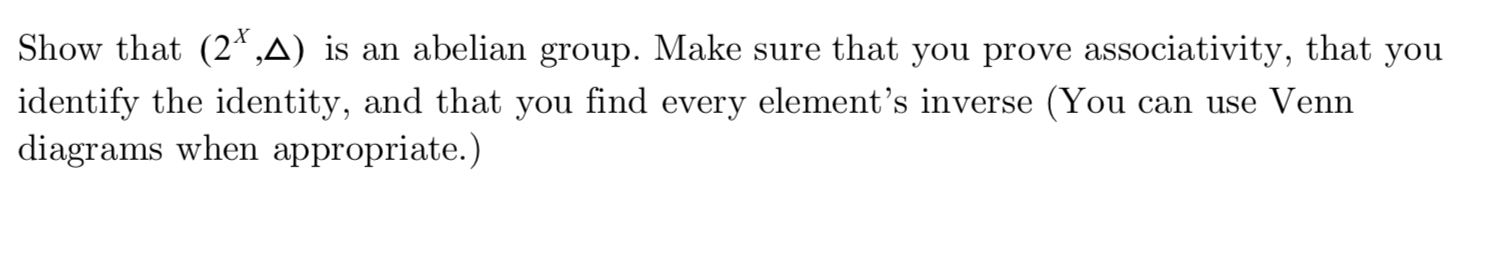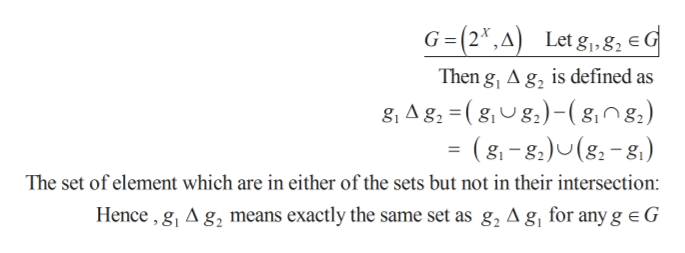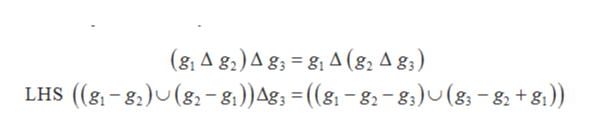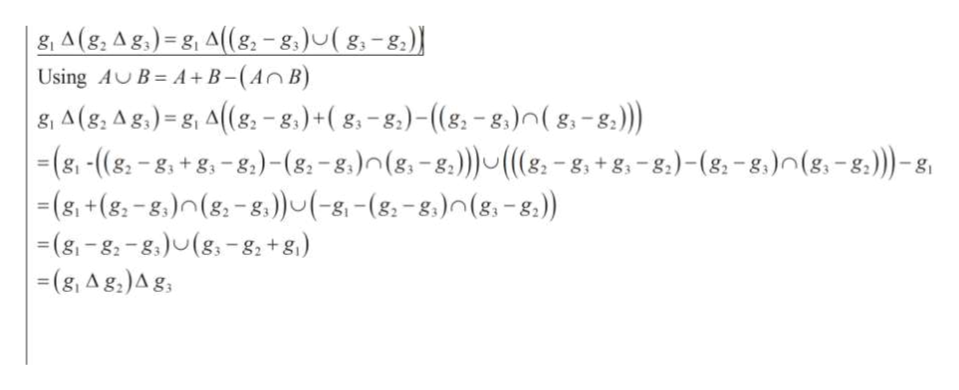Show that (2*,A) is an abelian group. Make sure that you prove associativity, that youidentify the identity, and that you find every element's inverse (Youdiagrams when appropriate.)can use Venn

Questionhelp_outlineImage TranscriptioncloseShow that (2*,A) is an abelian group. Make sure that you prove associativity, that you identify the identity, and that you find every element's inverse (You diagrams when appropriate.) can use Venn fullscreen
Step 1

First to prove that the given group is abelian.

Here in the given group

G is the set of all subsets of a set X, so G can be present here as a power set of X.

Cardinality of the group depend on the set G, if X is finite then group G is finite otherwise it is infinite group.help_outlineImage TranscriptioncloseG(2,A) Let g, 8, eG Then g, A g, is defined as 8, A g, (gg)-(g,0g.) = (8,-8)U(g-8) The set of element which are in either of the sets but not in their intersection: Hence,g, A g2 means exactly the same set as g, A g, for any g e G fullscreen
Step 2

In order to prove associativityhelp_outlineImage Transcriptionclose(g1 A g)A g 8, A (g2 A g3) LHS ((8-8) (8-81))Ag = ((8-8-gs)U (g-8 +8) fullscreen
Step 3

Now, looking for the RHS...help_outlineImage Transcriptionclose|g A (g, A g,) = g, A((8-8)u(8-8) A+B-(An B) Using AB , A(g, Ag,) = g, A(g -8.)+( g-8)- ((g-g.) ( g, - s) |(g, -(8-8, +8,-8,) - (8. -8)n(8,-8) ((&-8, +8,-8)- (8-8)n(8,-8)-g (&, +(8-8,)(g-8))(-g-(8-8)n (g,-8) (g-g-8(g -8 +g) (g, Ag,)Ag fullscreen

Want to see the full answer?

See Solution

Want to see this answer and more?

Our solutions are written by experts, many with advanced degrees, and available 24/7

See Solution
Tagged in

Math